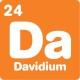# What's new## 4 equal rectangles

, 13 September 2023

Difficulty: Fun

The big rectangle below is made from 4 small rectangles. All the small rectangles are equal, meaning that they have the same size and shape.

If the small rectangle’s short side is 8 centimetres, what is the length (long side) of the bigger rectangle?Scroll down or click for a hint, or the answer!## Brainteaser hint

Remember that all the small rectangles have the same dimensions.
Where else can you label “8 cm” on the diagram?The length (long side) of the bigger rectangle is 32 centimetres.

The key to solving this geometry problem lies in the title: 4 equal rectangles. We know from the problem that the short side of all the small rectangles is 8 centimetres. This includes the middle rectangles lying in the centre, if we label their short sides as 8 centimetres we get:Now we know the long side of the small rectangles is 8 + 8 = 16 centimetres. Since the small rectangles are all equal, this dimension must be true for all of them.

The next step is to add up all the lengths along the long side of the bigger rectangle:

Bigger rectangle width = short side of small rectangle + long side of small rectangle + short side of small rectangle

= 8 + 16 + 8

= 32 centimetres.Categories:

## Similar posts

1.The length of the rectangle is 32 cms, and the width is 16 cms.

2.I agree with Mark, width is usually the shorter of the two distances, whether the shape is displayed in portrait or landscape.

3.Since height or length are not specified, then width is open to interpretation. You could see it as a window, so horizontal width, or as a tennis court, so narrow width. Good observation for Mark and Norman.

4.Thanks for the keen edits! We’ve updated the problem to use length and long side so there’s no confusion.

5.Thank you for making the correction. However, you have not changed the original question on the opening page. You still ask, ‘ . . . what is the width (of) the big rectangle?’
It should be ‘. . . what is the length of the bigger rectangle.’ Or, if you prefer, ‘. . . what is the length (long side) of the bigger rectangle?’

1.updated!